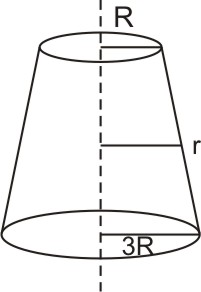Q

# Need explanation for: A uniformly tapering conical wire is made from a material of Young’s modulus Y and has a normal, unextended length L. The radii, at the upper and lower ends of this conical wire, have values R and 3R, respectively. The upp

A uniformly tapering conical wire is made from a material of Young’s modulus Y and has a normal, unextended length L.  The radii, at the upper and lower ends of this conical wire, have values R and 3R, respectively.  The upper end of the wire is fixed to a rigid support and a mass M is suspended from its lower end.  The equilibrium extended length, of this wire, would equal :

• Option 1)• Option 2)• Option 3)• Option 4)738 Views

As we learnt in

Young Modulus -

Ratio of normal stress to longitudnal strain

it denoted by Y

- wherein

F -  applied force

A -  Area

-  Change in lenght

l - original lengthNow,

Correct option is 2.

Option 1)This is an incorrect option.

Option 2)This is the correct option.

Option 3)This is an incorrect option.

Option 4)This is an incorrect option.

Exams
Articles
Questions